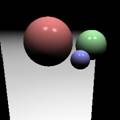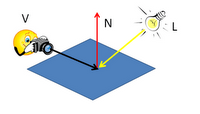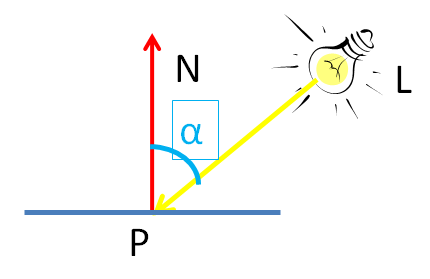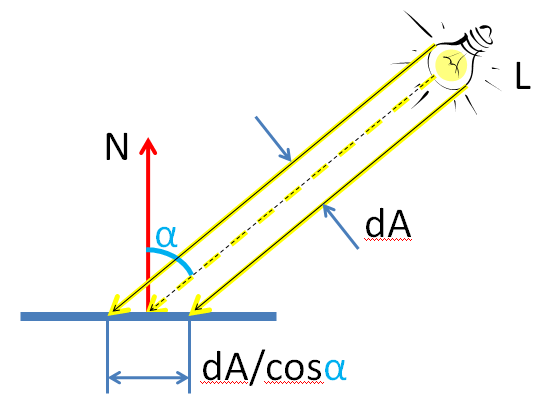# Modelos de iluminación: LightAn animated scene is illuminated by a global properties (Ambient Light) and by different points of light (Spotlight) that emulate many other types of “lamps”. Mathematical calculations performed with these parameters, applied to the geometry which defines the scene, are associated with the concept of “Modelos de iluminación“.

Rooms, Lambert, Fressnell, Minnaert, Toon, Oren-Nayar, Toon etc are some of the names that some major lighting models are typically referenced.

It is necessary to understand in depth models for use in artistic creation tools synthetic image, but we recommend a basic knowledge to understand how images are formed to anticipate results in application.Phon model is mathematically simple and gives very correct images. Models based on ray tracing enable brighter and realistic images in certain fields of application. Other concepts such as radiant energy of the bodies can address the problem from very different perspectives they bring new features to images.

Exploring some basics can give us a different view of the action produced by light sources on an object in the scene. The idea of ​​what a gloss or why allow a shadow occurs gradually introduce the basic mathematical model effortlessly. We go deeper into each of these items always from the simplest ideas, our aim is to understand or get a rough idea of ​​how each calculation is determined on the most basic models.

## Points of Light

Each light spot (L) defined with different parameters:

• intensity
• color
• Minimum and maximum ranges
• attenuation model intensity
• directionality of the beam …

This variety of parameters allows it to adapt to behavior that is intended to emulate in every type of lamp built into the imaging software synthesis.The illumination intensity at a point in the scene, depends on the positions of Camera ( V.. ) and spotlights (L) with respect to the surfaces.

At each point of a surface is a direction orthogonal (perpendicular) to the surface that can be represented by the direction (N) .

A plane for example has at all points of its surface the same “normal direction”, are parallel, while in the case of a sphere all passing through its center perpendicular, and cover all possible directions in space.

We have three basic points of our basic alphabet to begin to link the objects and lights to the image obtenddremos “render” Scene, namely, when the program converts the objects and data in a single image or an entire animation.

## Light IntensityThe light intensity at each point depends on various factors. Of course, the first factor is the intensity lamp having (power of the bulb), but there are other factors that condition.

The greater the distance between the light and the object will lower the contribution of light that point to that object.

Another factor will significantly influence the direction in which the light is received.

An important angle appears on this model, which form the beam (L) with normal (N) to the surface (angle alpha).If we imagine a light beam as a cylinder of the illumination spot can understand the dependence between the alpha angle and intensity of light that reaches a point.

The cylinder has a thickness and consequently covers an area (dA) that strike the surface becomes the illuminated area. Its size depends on the angle alpha.

We can see the effect described home: if inclined flashlight, its ground clearance changes shape and its intensity decreases with distance.

The new illuminated area of ​​the cylinder is divided by a function, cosine of alpha. This simple equation shows how radiant energy is distributed (light) on a surface, depending on its inclination. A greater illuminated surface, lower intensity, then decreases with increasing alpha “light quantity” reaching each point.

What matters is that the illuminated area changes with a mathematical expression that relates us with the intensity angle that is received on the surface, therefore vary depending on where we place these points in the scene. Near or far?? Do Higher or lower? With varying intensity (power) each light?